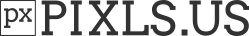# RA and DEC in ZWO ASI Fits Header

I often have the case that Siril cannot detach a plate, although the correct RA and DEC are entered in the Fits header (ZWO ASI).

I found the reason:

Two coordinates are entered in the ZWO ASI Fits header
RA = 312.993 / Object Right Ascension in degrees
DEC = 31.9068 / Object Declination in degrees

CTYPE1 = ‘RA—TAN-SIP’ / TAN (gnomic) projection + SIP distortions
CTYPE2 = ‘DEC–TAN-SIP’ / TAN (gnomic) projection + SIP distortions
CRVAL1 = 312.121179008 / RA of reference point
CRVAL2 = 33.7346625176 / DEC of reference point
CRPIX1 = 223.516437531 / X reference pixel
CRPIX2 = 420.223754883 / Y reference pixel

The coordinates in the image center are these (identical to the solution of nova.astrometry.net)
RA 312.993 / DEC 31.9068

Unfortunately, Siril uses coordinates CRVAL1/CRVAL2 and therefore does not find a plate solution, especially for wide-field images

// image_format_fits.c
fits_read_key(fit->fptr, TDOUBLE, “CRVAL1”, &(fit->wcsdata.crval), NULL, &status);
fits_read_key(fit->fptr, TDOUBLE, “CRVAL2”, &(fit->wcsdata.crval), NULL, &status);

//Camera ZWO ASI183MC 5.496x3.672 written by ZWO ASIAIR

SIMPLE = T / file does conform to FITS standard
BITPIX = 16 / number of bits per data pixel
NAXIS = 2 / number of data axes
NAXIS1 = 5496 / length of data axis 1
NAXIS2 = 3672 / length of data axis 2
EXTEND = T / FITS dataset may contain extensions
COMMENT FITS (Flexible Image Transport System) format is defined in ‘Astronomy
COMMENT and Astrophysics’, volume 376, page 359; bibcode: 2001A&A…376…359H
BZERO = 32768 / offset data range to that of unsigned short
BSCALE = 1 / default scaling factor
CREATOR = ‘ZWO ASIAIR’ / Capture software
OFFSET = 10 / camera offset
XORGSUBF= 0 / Subframe X position in binned pixels
YORGSUBF= 0 / Subframe Y position in binned pixels
FOCALLEN= 129 / Focal length of telescope in mm
EGAIN = 0.361999988555908 / Electronic gain in e-/ADU
XBINNING= 1 / Camera X Bin
YBINNING= 1 / Camera Y Bin
CCDXBIN = 1 / Camera X Bin
CCDYBIN = 1 / Camera Y Bin
XPIXSZ = 2.40000009536743 / pixel size in microns (with binning)
YPIXSZ = 2.40000009536743 / pixel size in microns (with binning)
IMAGETYP= 'Light ’ / Type of image
EXPOSURE= 60. / Exposure time in seconds
EXPTIME = 60. / Exposure time in seconds
CCD-TEMP= 19.2999992370605 / sensor temperature in C
RA = 312.993 / Object Right Ascension in degrees
DEC = 31.9068 / Object Declination in degrees
DATE-OBS= ‘2021-06-26T23:08:59.635’ / Image created time
INSTRUME= ‘ZWO ASI183MC’ / Camera model
BAYERPAT= 'RGGB ’ / Bayer pattern
GAIN = 200 / Gain Value
TELESCOP= ‘EQMod Mount’ / Telescope name
CTYPE1 = ‘RA—TAN-SIP’ / TAN (gnomic) projection + SIP distortions
CTYPE2 = ‘DEC–TAN-SIP’ / TAN (gnomic) projection + SIP distortions
CRVAL1 = 312.121179008 / RA of reference point
CRVAL2 = 33.7346625176 / DEC of reference point
CRPIX1 = 223.516437531 / X reference pixel
CRPIX2 = 420.223754883 / Y reference pixel
CD1_1 = 0.000807569282086 / Transformation matrix
CD1_2 = 0.00418054387455 / no comment
CD2_1 = -0.00417444284354 / no comment
CD2_2 = 0.000809792917224 / no comment
A_ORDER = 2. / Polynomial order, axis 1
B_ORDER = 2. / Polynomial order, axis 2
AP_ORDER= 2. / Inv polynomial order, axis 1
BP_ORDER= 2. / Inv polynomial order, axis 2
A_0_0 = 0. / no comment
A_0_1 = 0. / no comment
A_0_2 = -3.25240106779E-07 / no comment
A_1_0 = 0. / no comment
A_1_1 = 6.0794245259E-07 / no comment
A_2_0 = 2.61332074741E-06 / no comment
B_0_0 = 0. / no comment
B_0_1 = 0. / no comment
B_0_2 = 1.11192069938E-06 / no comment
B_1_0 = 0. / no comment
B_1_1 = 2.39138889379E-06 / no comment
B_2_0 = -9.69984828717E-08 / no comment
AP_0_0 = -0.000456247442657 / no comment
AP_0_1 = -3.32926651844E-07 / no comment
AP_0_2 = 3.24113136033E-07 / no comment
AP_1_0 = -4.99420259789E-06 / no comment
AP_1_1 = -6.0232632373E-07 / no comment
AP_2_0 = -2.59430009291E-06 / no comment
BP_0_0 = -0.000279764680655 / no comment
BP_0_1 = -6.52274365771E-07 / no comment
BP_0_2 = -1.10734330604E-06 / no comment
BP_1_0 = 5.37876957752E-07 / no comment
BP_1_1 = -2.37993967797E-06 / no comment
BP_2_0 = 9.64151604334E-08 / no comment
IMAGEW = 1372. / Image width, in pixels.
IMAGEH = 916. / Image height, in pixels.
END

CRVAL1 and CRVAL2 are standard for astrometry. Not RA and DEC I believe.

Also, for reading fits header astrometry we use the wcslib library that was written by the guys who created the wcs keywords.

What’s that? The image size in pixel is that:

Yes, indeed

Mark Calabretta told me that IMAGEW and IMAGEH was kind of deprecated too. This is why I don’t use it.

Also, CRVAL1 and CRVAL2 are the center of the image in alpha, dec coordinates. And this is what we need to start platesolving (with resolution).

OK I think I understand.
ASIAIR downscale the image before platesolving (by 4). This is why IMAGEW and IMAGEH are different to the image size.
Then, crpix values are not in the center of image as we generally do. So we have to take into account this possibility.

@jfried could you share the image please

it’s not the best image but a good sample

Light_NGC6974_60.0s_Bin1_gain200_20210627-010859_19.3C_0088.fit

Screenshot (same in v 0.99.10.1)
Siril found a solution for manual coordinates from Fits Header
RA = 312.993 → 20h 52min
DEC = 31.9068 → 31° 54min

and with Button “Get Metadata From Image”

This is fixed now.

However there is a crash with image from ASIAIR in some cases. Need to find if it is a bug from us, or from the wcslib.

I saw that you adopted the RA and DEC tagsThank you very much for the fast service1 Like